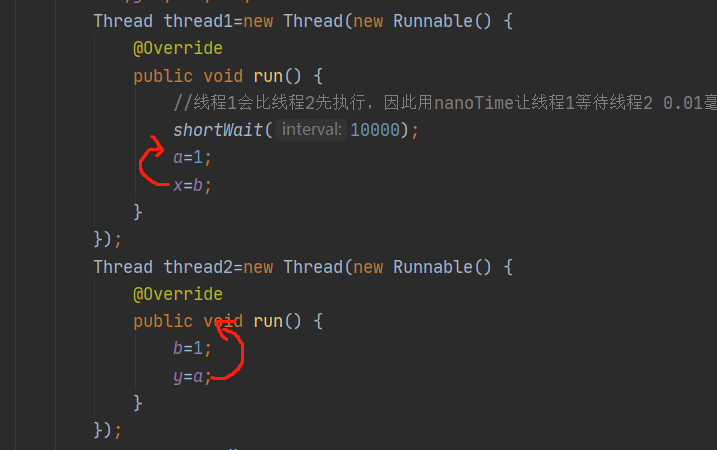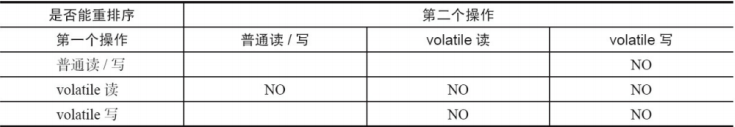# 指令重排序、内存屏障很难？看完这篇你就懂了！Java鱼仔

# （一）什么是指令重排序

Java 中的指令重排序有两次，第一次发生在将字节码编译成机器码的阶段，第二次发生在 CPU 执行的时候，也会适当对指令进行重排。

# （二）复现指令重排序

public class VolatileReOrderSample {    //定义四个静态变量    private static int x=0,y=0;    private static int a=0,b=0;    public static void main(String[] args) throws InterruptedException {        int i=0;        while (true){            i++;            x=0;y=0;a=0;b=0;            //开两个线程，第一个线程执行a=1;x=b;第二个线程执行b=1;y=a            Thread thread1=new Thread(new Runnable() {                @Override                public void run() {                    //线程1会比线程2先执行，因此用nanoTime让线程1等待线程2 0.01毫秒                    shortWait(10000);                    a=1;                    x=b;                }            });            Thread thread2=new Thread(new Runnable() {                @Override                public void run() {                    b=1;                    y=a;                }            });            thread1.start();            thread2.start();            thread1.join();            thread2.join();            //等两个线程都执行完毕后拼接结果            String result="第"+i+"次执行x="+x+"y="+y;            //如果x=0且y=0，则跳出循环            if (x==0&&y==0){                System.out.println(result);                break;            }else{                System.out.println(result);            }        }    }    //等待interval纳秒    private static void shortWait(long interval) {        long start=System.nanoTime();        long end;        do {            end=System.nanoTime();        }while (start+interval>=end);    }}# （三）通过什么方式禁止指令重排序？

Volatile 通过内存屏障可以禁止指令重排序，内存屏障是一个 CPU 的指令，它可以保证特定操作的执行顺序。

JMM 针对编译器制定了 Volatile 重排序的规则：## 评论